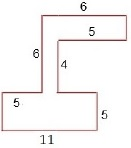# Perimeter of a Polygon Online Quiz

Following quiz provides Multiple Choice Questions (MCQs) related to Perimeter of a Polygon. You will have to read all the given answers and click over the correct answer. If you are not sure about the answer then you can check the answer using Show Answer button. You can use Next Quiz button to check new set of questions in the quiz.Q 1 - If the side lengths of a polygon are 10 m, 11 m, 12 m, 13 m, 14 m, 16 m what is its perimeter?

A - 72 m

B - 72 cm

C - 76 m

D - 78 m

### Explanation

The perimeter of the polygon is

10 m + 11 m + 12 m + 13 m + 14 m + 16 m = 76 m

Q 2 - If the length of side of a regular polygon of 15 sides is 11 feet, then find its perimeter.

A - 165 feet

B - 165 m

C - 175 feet

D - 155 feet

### Explanation

Perimeter of the regular polygon of 15 sides of side

11 feet = 15 × 11 = 165 feet

Q 3 - If the length of side of a regular polygon of 12 sides is 9 cm, then find its perimeter.

A - 96 cm

B - 96 m

C - 108 m

D - 108 cm

### Explanation

Perimeter of the regular polygon of 12 sides of

side 9 cm = 12 × 9 = 108 cm

Q 4 - If the side lengths of a polygon are 7 cm, 8 cm, 9 cm, 10 cm, 15 cm, what is its perimeter?

A - 49 m

B - 49 cm

C - 48 cm

D - 48 m

### Explanation

The perimeter of the polygon is

7 cm + 8 cm + 9 cm + 10 cm + 15 cm = 49 cm

Q 5 - If the side lengths of a polygon are 10 cm, 11 cm, 9 cm, 10 cm, 12cm, 16 cm what is its perimeter?

A - 68 cm

B - 66 cm

C - 68 m

D - 66 m

### Explanation

The perimeter of the polygon is

10 cm + 11 cm + 9 cm + 10 cm + 12 cm + 16 cm = 68 cm

Q 6 - If the length of side of a regular polygon of 16 sides is 8 m, then find its perimeter.

A - 124 m

B - 126 m

C - 128 m

D - 128 cm

### Explanation

Perimeter of the regular polygon of 16 sides of

side 8 m = 16 × 8 = 128 m

Q 7 - If the length of side of a regular polygon of 8 sides is 13 units, then find its perimeter.

A - 108 units

B - 104 cm

C - 104 m

D - 104 units

### Explanation

Perimeter of the regular polygon of 8 sides of

side 13 units = 8 × 13 = 104 units

Q 8 - Find the perimeter of the following polygon.A - 38 m

B - 38 units

C - 38 cm

D - 39 units

### Explanation

The perimeter of the polygon is

6 + 9 + 2 + 2 + 6 + 4 + 2 + 7 = 38 units

Q 9 - Find the perimeter of the following polygonA - 40 units

B - 40 m

C - 42 units

D - 40 cm

### Explanation

The perimeter of the polygon is

8 + 2 + 5 + 5 + 2 + 4 + 5 + 11 = 42 units

Q 10 - Find the perimeter of the following polygon.A - 54 units

B - 56 m

C - 56 units

D - 56 cm

### Explanation

The perimeter of the polygon is

5 + 6 + 6 + 2 + 5 + 4 + 5 + 5 + 11 + 5 = 54 units

perimeter_of_polygon.htm